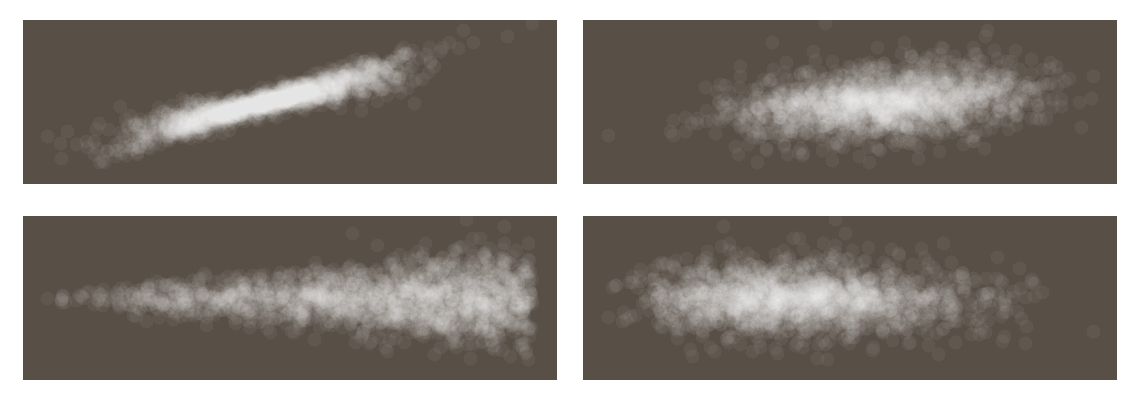# Can scatter plots be used for sensitivity analysis?

subject area
in shortprerequisite knowledge

Scatter plots of the input parameters against the model output are one of the most straightforward and most versatile tools for qualitative global sensitivity analysis. Indeed, most quantitative sensitivity measures attempt to preserve the information content provided by scatter plots in a condensed format [Saltelli, 2008]. The advantage of the graphical analysis is that it provides an overview of the model sensitivities, independent of the degree of non-linearity [Straub, 2019]. Scatter plots are very helpful in illustrating the dependency between an input quantity and the model output. The clearer the discernible shape/pattern in a scatter plot, the more important the respective parameter is in the model.

Scatter plots are usually based on samples from a Monte Carlo simulation. Samples of the model output are plotted on the vertical axis of the scatter plot. The corresponding samples of the input are drawn on the horizontal axis. A separate scatter plot is needed for each input parameter if the model has multiple input parameters.

Even though these bidimensional scatter plots are generally very helpful and highly informative, in special cases, they may not be able to identify an influential factor. This can happen if higher-dimensional interactions of the model input parameters with the model output exist. A general limitation of scatter plots is that interactions of groups of input parameters with the model output cannot be visualized by means of (two-dimensional) scatter plots.

## References

[Saltelli, 2008] Saltelli, Andrea, et al: Global sensitivity analysis: the primer. John Wiley & Sons, 2008.

[Straub, 2019]  Straub, Daniel: Lecture Notes in Engineering Risk Analysis. Technische Universität München, 2019.